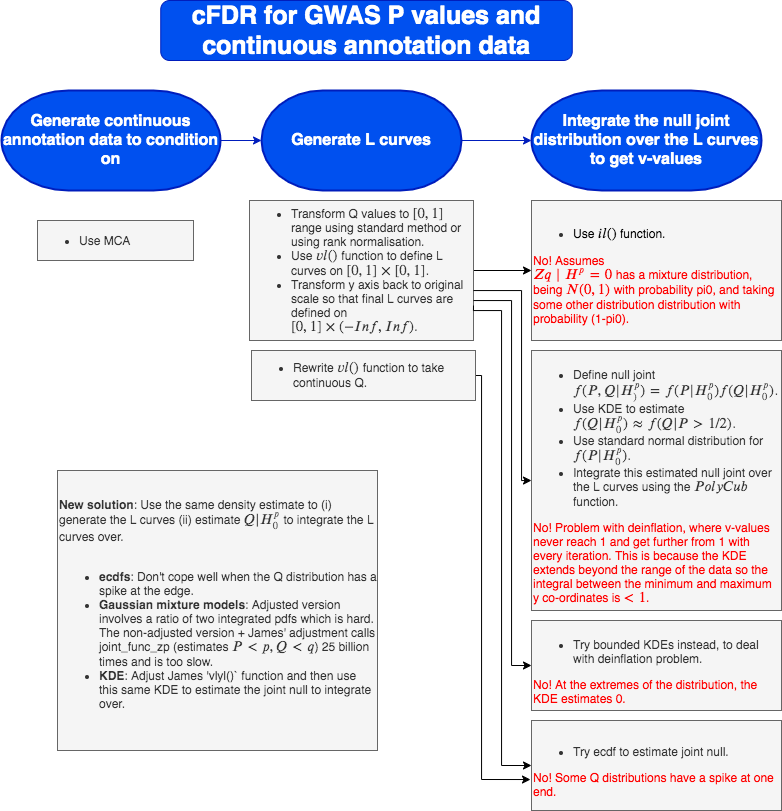### 1. Tying up loose ends

#### Comparing mixtools and mclust for density estimation using GMM

• mclust::densityMclust takes on average 10 seconds for 50,000 data points.

• mixtools::normalmixEM takes on average 24 seconds for 50,000 data points and requires specification of how may Gaussians.

#### Estimating $$P(P\leq p|Q\leq q)$$ (denominator in cfdr)

At the moment I am using:

$\begin{equation} P(P\leq p|Q\leq q) = \dfrac{P(P\leq p, Q\leq q)}{P(Q\leq q)} \end{equation}$

where $$P(Q\leq q)$$ cancels with part of the adjustment factor on the numerator.

$$P(P\leq p, Q\leq q)$$ is then estimated using the joint_func_zq function.

For each (p,q) pair…

• joint_func_zq is called nt=5000 times (length of xtest) to generate the cfdr curve to estimate ccut.

• cfdr curves are then fit for each entry in yval2. Meaning that for each (p,q) pair, joint_func_zq is called nv=1000 * nt=5000 times (length of yval2 * length of xtest).

That means for each (p,q) pair, joint_func_zq is called 25,000,000,000 times!! It takes 4.6 milliseconds to call but in my sapply loop, it takes 14 seconds to fit to nt=5000 x coordinates.

Alternatively, Chris has suggested estimating this conditional probability directly using a univariate mixture of normals. I.e. we fit it to all (SIGNED) zp values and then do something else… We use signed z scores because then hopefully all the means are approximately 0. –> may come back to this later.

### 2. Taking a step back

I summarise the methods I have explored so far.The idea now is to use the same density estimate to (i) generate the L curves (ii) estimate $$Q|H_0^p$$ to integrate the L curves over.

### vl vrs vlyl

I investigate using KDEs for the L curves and for estimating the null to integrate. Using James’ standard code in the cfdr package, I observe that the L curves are pretty different when using vlyl() compared with vl(). [Note that vlyl() includes the adjustment so need to specify adj=TRUE in the vl() function].